# A Solow-Malthus Model of Ancient Economies¶

## 1: The Model Equations in a Nutshell ¶

Income and output $Y$ as a function of the capital stock $K$, the efficiency of labor $E$, the labor forcer $L$ and the capital elasticity of income $\alpha$:

(1.1) $Y = K^{\alpha}(EL)^{1-\alpha}$

Capital-stock growth $g_k$ as a function of the savings-investment share $s$, income and output $Y$, and the depreciation rate $\delta$:

(1.2) $\frac{dK/dt}{K} = \frac{d\ln(K)}{dt} = g_k = \frac{sY}{K} - \delta$

Efficiency of labor growth $g$ as a function of the growth rate $h$ of the useful-ideas stock, and of resource scarcity generated by labor force growth $n$ in conjunction with an importance-of-ideas-relative-to-resources parameter $\gamma$:

(1.3) $\frac{dE/dt}{E} = \frac{d\ln(E)}{dt} = g = h - \frac{n}{\gamma}$

Income per worker (and, implicitly, per capita):

(1.4) $y = \frac{Y}{L}$

Labor force growth n as a function of a population growth elasticity with respect to Malthusian "fitness" $\beta$, of income devoted to Malthusian "fitness" $y/\phi$ (where $\phi$ captures relative expenditure on "luxuries") and of the zero population growth "subsistence" level of income devoted to Malthusian "fitness" $y^{sub}$:

(1.5) $\frac{dL/dt}{L} = \frac{d\ln(L)}{dt} = n = \beta \left( \frac{y}{\phi y^{sub}}-1 \right)$

And, for convenience, to make some equations simpler, define the capital-intensity elasticity of income as:

(1.6) $\theta = \frac{\alpha}{1-\alpha}$

and define capital intensity as:

(1.7) $\kappa = \frac{K}{Y}$

with its proportional rate of growth:

(1.8) $\frac{d\ln(\kappa)}{dt} = g_\kappa = g_k - g_y$

Sometimes we will replace the efficiency-of-labor equation (1.3) by:

(1.3') $\frac{dE/dt}{E} = \frac{d\ln(E)}{dt} = g$

and the labor-force and population growth equation (1.4) by:

(1.4') $\frac{dL/dt}{L} = \frac{d\ln(L)}{dt} = n$

These replacements transform the system from Solow-Malthus back to the standard Solow Growth Model.

## 2.1: The Basic Solow Setup ¶

Here, as always, we do what economists do: ruthlessly simplify. People control resources, and use this resources in ways that respond to incentives, and so the societal system moves forward. Economists write down very simple equations describing this: behavioral relationships (how people act), equilibrium conditions (what people's interactions entail), and identities (adding-up conditions. From those they derive how the societal system should have behaved or be behaving. It goes wrong. So economists then go back and complicate the model, and see what the complication entails. They then iterate, until they believe that they have gotten close enough to say that the final-stage model is a good-enough one.

Thus we start with the Solow Growth Model basic setup: income (and output) $Y$ as a function of the capital stock $K$, the labor force $L$, the efficiency of labor $E$, and the decreasing-returns parameter $\alpha$ are determined according to (1.1). Growth rates of the capital stock, the efficiency of labor, and of the labor force are determined according to (1.2), (1.3'), and (1.4'). These entail that the growth rate of $Y$, income and output, is: as functions of the savings-investment rate $s$, the population and labor force growth rate $n$, the efficiency-of-labor growth rate $g$, and the depreciation rate $\delta$:

(2.1) $\frac{d\ln(Y)}{dt} = g_{n+y} = \alpha g_k + (1-\alpha)n + (1-\alpha)g$

### 2.2: Determining the Equilibrium Capital-Output Ratio $\kappa^*$ ¶

Now fix the parameters $\alpha, \beta, s, g$, and look for a situation in which $g_\kappa = 0$: in which the capital stock $K$ and production $Y$ are growing at the same rate so that the capital-output ratio $g_\kappa$ is constant.

Substituting into (2.1):

(2.2) $g_\kappa = g_k - \left( \alpha g_k + (1-\alpha)n + (1-\alpha)g \right)$

(2.3) $g_\kappa - g_{y+n} = (1-\alpha)\left( g_k - n - g \right)$

(2.4) $g_\kappa = (1-\alpha)\left( \frac{sY}{K} - \delta - n - g \right)$

(2.5) $n + g + \delta = \frac{sY}{K}$ whenever $g_\kappa = 0$

(2.6) $\kappa = \frac{K}{Y} = \frac{s}{n + g + \delta}$ whenever $g_\kappa = 0$

So we define the steady-state growth equilibrium capital-output ratio:

(2.7) $\kappa^* = \left( \frac{K}{Y} \right)^* = \frac{s}{n + g + \delta}$

### 2.3: Determining Steady-State Growth-Path Production per Worker ¶

Recall:

(2.8) $\ln(Y) = \alpha\ln(K) + (1-\alpha)\left(\ln(E)+\ln(L)\right)$

from that derive:

(2.9) $\ln(Y) = \alpha\left(\ln(\kappa) + \ln(Y) \right) + (1-\alpha)\left(\ln(E)+\ln(L)\right)$

(2.10) $(1-\alpha)\ln(Y) = \alpha\ln(\kappa) + (1-\alpha)\left(\ln(E)+\ln(L)\right)$

(2.11) $\ln(Y) = \left( \frac{\alpha}{1-\alpha} \right)\ln(\kappa) + \ln(L) + \ln(E)$

(2.12) $\ln \left( \frac{Y}{L} \right) = \left( \frac{\alpha}{1-\alpha} \right)\ln(\kappa) + \ln(E)$

This is where we want to make things simpler by using $\theta = \alpha/(1-\alpha)$:

(2.13) $\ln \left( \frac{Y}{L} \right) = \theta\ln(\kappa) + \ln(E)$

And so we define steady-state growth-path production-per-worker as:

(2.14) $\ln \left( \frac{Y}{L} \right)^* = \theta \ln(\kappa^*) =+ \ln(E)$

(2.15) $\left(\frac{Y}{L}\right)^* = \left(\kappa^*\right)^\theta E$

(2.16) $\left(\frac{Y}{L}\right)^* = \left( \frac{s}{n+g+\delta} \right)^\theta E$

(2.17) $\frac{d}{dt} \left(\frac{Y}{L}\right)^* = g$

Along the steady-state growth path, production per worker $Y/L$, capital per worker $K/L$, and the efficiency of labor $E$ both grow at the proportional rate $g$; the population and labor force $L$ grows at the proportional rate $n$; total production $Y$ and the capital stock $K$ grow at the proportional rate $n + g$; and the capital-output ratio $\kappa = K/Y$ is constant.

An economy well-modeled by this Solow Growth Model heads for a balanced-growth equilibrium path on which there is a constant balanced-growth capital-output ratio $\kappa^* = s/(n+g+\delta)$, a constant growth rate $g$ of income (and production) and the capital stock per worker, a constant growth rate $n$ of the labor force, and a constant growth rate $n + g$ of total societal income (and production) and of the total capital stock.

How fast does this economy head for its balanced-growth equilibrium path? Recall (2.4):

(2.4) $\frac{1}{\kappa}\frac{d\kappa}{dt} = g_\kappa = (1-\alpha)\left( \frac{s}{\kappa} - (n+g+\delta) \right)$

(2.18) $\frac{d\kappa}{dt} = (1-\alpha)s -(1-\alpha)(n+g+\delta)\kappa$

(2.19) $\frac{d\kappa}{dt} = -(1-\alpha)(n+g+\delta)(\kappa - \kappa^*)$

We have exponential convergence with a $1/e$ time of $1/[(1-\alpha)(n+g+\delta)]$.

But this is not the ancient economy. In the ancient economy $g = 0$, or is very close. The model is unsatisfactory in that it does not provide an explanation for why $g$ is near zero. Hence we complicate the model by introducing Malthusian elements.

## 3: Population, Resource Scarcity, and the Efficiency of Labor ¶

### 3.1: Determinants of the Efficiency of Labor ¶

Now let's complicate the determinants of the efficiency of labor. Let's revert to (1.3): efficiency of labor growth $g$ is a funciton of the rate $h$ at which economically useful ideas are generated, but also of the rate of population and labor force growth $n$ because a higher population makes resources per capita scarce, as determined by an effect-of-resource scarcity parameter $\gamma$:

(1.3) $\frac{dE/dt}{E} = \frac{d\ln(E)}{dt} = g = h - \frac{n}{\gamma}$

Thus:

(3.1) $\frac{d}{dt} \left(\frac{Y}{L}\right)^* = 0$ whenever $h - \frac{n}{\gamma} = 0$

(3.2) $n^{*mal} = \gamma h$ is the population growth rate at which $\frac{d}{dt} \left(\frac{Y}{L}\right)^* = 0$

When population is growing at the rate $n^{*mal}$, the efficiency of labor—and thus the steady-state growth-path level of production per worker $Y/L$—is constant.

### 3.2: Determinants of Population and Labor Force Growth ¶

Now let's revert to (1.5): make the rate of growth of the population and labor force depend on the level of prosperity $y = Y/L$; on the "subsistence" standard of living for necessities $y^{sub}$; and also on the fraction $1/\phi$ of production that is devoted to necessities, not conveniences and luxuries, and thus enters into reproductive and survival fitness:

(1.5) $\frac{dL/dt}{L} = \frac{d\ln(L)}{dt} = n = \beta \left( \frac{y}{\phi y^{sub}}-1 \right)$

Then for population to be growing at its Malthusian rate:

(3.3) $\gamma h = \beta \left(\frac{1}{\phi}\right) \left( \frac{y}{y^{sub}}- \phi \right)$

(3.4) $\frac{\phi \gamma h}{\beta} = \left( \frac{y}{y^{sub}}- \phi \right)$

(3.5) $\frac{y}{y^{sub}} = \phi \left( 1 + \frac{\gamma h}{\beta} \right)$

(3.6) $y^{*mal} = \phi y^{sub} \left( 1 + \frac{ n^{*mal}}{\beta}\right) = \phi y^{sub} \left( 1 + \frac{ \gamma h}{\beta}\right)$

### 3.3: The Full Equilibrium ¶

We can determine the log level $E$ of the efficiency of labor:

(3.7) $\ln(E) = \ln(H) - \frac{\ln(L)}{\gamma}$

Recall (24):

(2.16) $y^* = \left( \frac{s}{n+g+\delta} \right)^\theta E$

Then:

(3.8) $y^{*mal} = \left( \frac{s}{\gamma h +\delta} \right)^\theta E$

(3.9) $\ln(\phi) + \ln\left( y^{sub} \right) + \ln\left(1 + \frac{\gamma h}{\beta} \right) = \theta \ln(s) - \theta \ln(\gamma h +\delta) + \ln(E)$

(3.10) $\ln(y^{sub}) = \theta \ln(s) - \theta \ln(\gamma h +\delta) + \ln(H_t) - \frac{\ln(L_t)}{\gamma} - \ln(\phi) - \ln\left(1 + \frac{\gamma h}{\beta} \right)$

(3.11) $\frac{\ln(L_t)}{\gamma} = \theta \ln(s) - \theta \ln(\gamma h +\delta) + \ln(H_t) - \ln(\phi) - \ln( y^{sub}) -ln\left(1 + \frac{\gamma h}{\beta} \right)$

Thus the population and labor force in the full Malthusian equilibrium will be:

(3.12) $\ln(L_t^{*mal}) = \gamma \left[ \theta \ln(s) - \theta \ln(\gamma h +\delta) + \ln(H_t) - \ln(\phi) - \ln( y^{sub}) -ln\left(1 + \frac{\gamma h}{\beta} \right) \right]$

It might be worthwhile decomposing this into terms depending on: (1) the level and rate of growth of the stock of ideas; (2) the rule of law and thrift that drive investment; (3) luxuries (including urbanization and elites); and demography in form of sociological (and law-and-order) determinants of "subsistence":

(3.13) $\ln(L_t^{*mal}) = \gamma \left[ \ln(H_t) - \ln( y^{sub}) \right] + \gamma \theta \left( \ln(s) - \ln(\delta) \right) - \gamma \ln(\phi) + \left( - \gamma \theta \ln(1 + \gamma h/\delta) -\gamma ln\left(1 + \frac{\gamma h}{\beta} \right) \right)$

or:

(3.14) $L_t^{*mal} = \left[ \left( \frac{H_t}{y^{sub}} \right) \left( \frac{s}{\delta} \right)^\theta \left( \frac{1}{\phi} \right) \left[ \frac{1}{(1+\gamma h/\delta)^\theta} \frac{1}{(1+\gamma h/\beta)} \right] \right]^\gamma$

And recall the Malthusian equilibrium standard of living:

(3.8) $y^{*mal} = \phi y^{sub} \left( 1 + \frac{ \gamma h}{\beta}\right)$

Plus for the rate of population growth:

(3.2) $n^{*mal} = \gamma h$

Thus we have our equilibrium for the pre-industrial Malthusian economy:

• Start with the rate $h$ at which new economically-useful ideas are being generated and with the responsiveness $\beta$ of population growth to increased prosperity.

• From those derive the Malthusian rate of population growth: $n^{*mal} = \gamma h$

• Then the Malthusian standard of living is: $y^{*mal} = \phi y^{sub} \left( 1 + \frac{ \gamma h}{\beta}\right)$

The Malthusian equilibrium standard of living is:

1. The luxuries-and-conveniences parameter $\phi$, times
2. The sociologically-determined level of subsistence $y^{sub}$, times
3. The (small and constant) nuisance parameter $1 + \gamma h/\beta$ needed to generate average population growth $n^{*mal} = \gamma h$.

Production per worker and thus prosperity is determined by (a) true subsistence, (b) the wedge between prosperity and reproductive fitness produced by spending on conveniences and luxuries that do not impact reproductive success, and (c) the wedge above subsistence needed to generate population growth consonant with the advance of knowledge and population pressure's generation of resource scarcity.

At any date t, the Malthusian-equilibrium population is:

1. the current level $H_t$ of the valuable ideas stock divided by the (sociologically determined, by, for example western European delayed female marriage patterns, or lineage-family control of reproduction by clan heads) Malthusian-subsistence income level $y^{sub}$ consistent with a stable population on average, times

2. the ratio between the savings-investment rate $s$ and the depreciation rate $\delta$, raised to the parameter $\theta$ which governs how much an increase in the capital-output ratio raises income—with a higher $\theta$ the rule of law, imperial peace, and a culture of thrift and invetment matter more, and can generate "efflorescences"—times

3. one over the conveniences-and-luxuries parameter $\phi$—it drives a wedge between prosperity and subsistence as spending is diverted categories that do not affect reproduction, such as middle-class luxuries, upper-class luxuries, but also the "luxury" of having an upper class, and the additional conveniences of living in cities and having trade networks that can spread plagues—times

4. two nuisance terms near zero, which depend on how much the level of population must fall below the true subsistence level at which population growth averages zero to generate the (small) average population growth rate that produces growing resource scarcity that offsets the (small) rate of growth of useful ideas. all this

5. raised to the power $\gamma$ that describes how much more important ideas are than resources in generating human income and production.

(1) is the level of the stock of useful ideas relative to the requirements for subsistence. (2) depends on how the rule of law and the rewards to thrift and entrepeneurship drive savings and investment, and thus the divisio of labor. (3) depends on how society diverts itself from nutrition and related activities that aim at boosting reproductive fitness and, instead, devotes itself to conveniences and luxuries—including the "luxury" of having an upper class, and all the conveniences of urban life. (4) are constant, and are small. And (5) governs how productive potential is translated into resource scarcity-generating population under Malthusian conditions.

And the Malthusian-equilibrium population and labor force is growing at the rate $n^{*mal} = \gamma h$.

### 4: Malthusian Convergence ¶

Recall:

(1.5) $\frac{dL/dt}{L} = \frac{d\ln(L)}{dt} = n = \beta \left( \frac{y}{\phi y^{sub}}-1 \right)$

(2.14) $\ln(y) = \theta\ln(\kappa) + \ln(H) -\ln(L)/\gamma$

Substitute:

(4.1) $\frac{1}{L}\frac{dL}{dt} = \frac{d\ln(L)}{dt} = n = \beta \left( \frac{\kappa^\theta H L^{-\gamma}}{\phi y^{sub}}-1 \right)$

(4.2) $\frac{d\kappa}{dt} = -(1-\alpha)(h + (1-1/\gamma)n +\delta)\kappa + (1-\alpha)s$

Define population-adjusted-for-ideas $P$:

(4.3) $P = L H^{-\gamma}$

Then we have two state variables—capital-intensity $\kappa$, the capital-output ratio, and population-adjusted-for-ideas $P$. We have two dynamic equations: The rate of change of population-adjusted-for-ideas $P$ is a function of the capital-output ratio and itself:

(4.4) $\frac{1}{P}\frac{dP}{dt} = p = \beta \left( \frac{\kappa^\theta }{P^{1/\gamma} \phi y^{sub}}-1 \right) -h\gamma$

And the rate of change of capital-intensity $\kappa$ is a function of itself and of the rate of change of population-adjusted-for-ideas $P$:

(4.5) $\frac{d\kappa}{dt} = (1-\alpha)s -(1-\alpha)((1-1/\gamma)p + \gamma h +\delta)\kappa$

With the two parameters $\alpha$ and $\theta$ related by:

(4.6) $\theta = \frac{\alpha}{1-\alpha}$

(4.7) $\alpha = \frac{\theta}{1+\theta}$

And if we set:

(4.8) $P = \Pi P^{*mal}$

(4.9) $\frac{1}{\Pi}\frac{d\Pi}{dt} = p = \beta \left[ \Pi^{(-1/\gamma)} \kappa^\theta (s/(\delta + \gamma h))^{-\theta} (1 + \gamma h/\beta) -1 \right] - h \gamma$

Define:

(4.10) $\kappa = k \kappa^{*mal} = k (s/(\delta + \gamma h))$

Then:

(4.11) $\kappa^{*mal} \frac{dk}{dt} = \left[ (1-\alpha)s -(1-\alpha)((1-1/\gamma)p + \gamma h +\delta) k (s/(\delta + \gamma h)) \right]$

And:

(4.12) $\frac{1}{\Pi}\frac{d\Pi}{dt} = p = \beta \left[ \Pi^{(-1/\gamma)} k^\theta (1 + \gamma h/\beta) -1 \right] - h \gamma$

This is our system.

Linearize around $\Pi = k = 1$, $p = 0$:

(4.13) $\frac{dk}{dt} = -(1-\alpha)(1-1/\gamma)p -(1-\alpha)(\delta + \gamma h)(k - 1)$

(4.14) $\frac{d\Pi}{dt} = p = - h (\Pi - 1) + \theta (\beta + \gamma h)(k - 1)$

(4.15) $\frac{dk}{dt} = (1-\alpha)(1-1/\gamma)h (\Pi - 1) -(1-\alpha)\left[ (1-1/\gamma)\theta (\beta + \gamma h) + (\delta + \gamma h) \right](k - 1)$

###### Malthusian Convergence will be maintained at: https://github.com/braddelong/long-form-drafts/blob/master/malthusian_convergence.ipynb¶

In :
# DEFINING CLASS MALTHUSIAN
#
# kept in delong_classes
#
# in general use:
#    import delong_classes
#
#    m = delong_classes.malthusian
#
#    .__init__ :: initialize
#    .update :: calculate the next year's values
#    .gen_seq :: return time series of selected variable

import matplotlib.pyplot as plt
%matplotlib inline

import numpy as np

class malthusian:

"""
Implements the Malthusian Model with:

1. population growth
n =  β*(y/(ϕ ysub)-1)

2. growth of efficiency-of-labor
g = h-n/γ
"""
def __init__(self,
L = 1,               # initial labor force
E = 1/3,             # initial efficiency of labor
K = 3.0,             # initial capital stock

# determinants of n (population growth):
β = 0.025,           # responsiveness of population growth to increased prosperity.
ϕ = 1,               # luxuries parameter
ysub = 1,            # subsistence level

# determinants of g(efficiency-of-labor growth):
h = 0,               # rate at which useful ideas are generated
γ = 2.0,             # effect-of-resource scarcity parameter

s = 0.15,            # savings-investment rate
α = 0.5,             # orientation-of-growth-toward-capital parameter
δ = 0.05,            # deprecation rate on capital parameter
):
self.L, self.E, self.K, self.h, self.γ, self.s, self.α, self.δ = L, E, K, h, γ, s, α, δ
self.β, self.ϕ, self.ysub = β, ϕ, ysub

# production (or output)
self.Y = self.K**self.α*(self.E*self.L)**(1-self.α)
self.y = self.Y/self.L

# capital-output ratio
self.κ = self.K/self.Y

# population growth
self.n = self.β*((self.y/(self.ϕ*self.ysub)) - 1)

# growth rate of efficiency-of-labor
self.g = self.h-self.n/self.γ

# store initial data
self.initdata = vars(self).copy()

def update(self):
"Calculate the next year's values"
# unpack parameters
K, s, Y, δ, L, n, E, g, α =self.K, self.s, self.Y, self.δ, self.L, self.n, self.E, self.g, self.α
β, ϕ, ysub, h, γ = self.β, self.ϕ, self.ysub, self.h, self.γ

#update variables
K = s*Y + (1-δ)*K
L = L*np.exp(n)
E = E*np.exp(g)
Y = K**α*(E*L)**(1-α)
y = Y/L
κ = K/Y
n = β*(y/(ϕ*ysub)-1)
g = h-n/γ

#store variables
self.K, self.s, self.Y, self.δ, self.L, self.n, self.E, self.g, self.α = K, s, Y, δ, L, n, E, g, α
self.κ, self.y = κ, y

def gen_seq(self, t, var = 'κ', init = True, log = False):
"Generate and return time series of selected variable. Variable is κ by default."

path = []

# initialize data
if init == True:
for para in self.initdata:
setattr(self, para, self.initdata[para])

for i in range(t):
path.append(vars(self)[var])
self.update()

if log == False:
return path
else:
return np.log(np.asarray(path))

def steady_state(self, disp = True):
"Calculate variable values in the steady state"
#unpack parameters
s, γ, h, δ, ϕ, ysub, β, α= self.s, self.γ, self.h, self.δ, self.ϕ, self.ysub, self.β, self.α

self.mal_κ = s/(γ*h+δ)
# malthusian rate of population growth
self.mal_n = γ*h
# malthusian standard of living
self.mal_y = ϕ*(ysub+γ*h/β)
self.mal_E = self.mal_y*((γ*h+δ)/s)**(α/(1-α))

if display == True:
return(f'steady-state capital-output ratio κ: {self.mal_κ:.2f}')
return(f'Malthusian rate of population growth n: {self.mal_n: .2f}')
return(f'Malthusian standard of living y: {self.mal_y:.2f}')
return(f'steady-state efficiency-of-labor E: {self.mal_E:.2f}')
else:
return(self.mal_κ,self.mal_n,self.mal_y,self.mal_E)

In :
# TESTING THE MALTHUSIAN CLASS
#
# No idea stock growth (h = 0)

import numpy as np
import matplotlib.pyplot as plt
%matplotlib inline

import delong_classes

T = 1000

m_base = delong_classes.malthusian(K=3.0)
m_base.scenario = "base scenario"
m_alt = delong_classes.malthusian(K=3.0)
m_alt.scenario = "alt scenario"

figcontents = {
(0,0):('κ','Capital-Output Ratio', False),
(0,1):('E','Efficiency of Labor', False),
(1,0):('L','Log Labor Force', True),
(1,1):('K','Log Capital Stock', True),
(2,0):('Y','Log Output', True),
(2,1):('y','Output-per-worker', False)
}

num_rows, num_cols = 3,2
fig, axes = plt.subplots(num_rows, num_cols, figsize=(12, 12))
for i in range(num_rows):
for j in range(num_cols):
for m in m_base, m_alt:
lb = f'{m.scenario}: initial κ = {m.initdata["κ"]:.2f}'
axes[i,j].plot(m.gen_seq(T, var = figcontents[i,j], log = figcontents[i,j]),'o-', lw=2, alpha=0.5, label=lb)
axes[i,j].set(title=figcontents[i,j])

#   global legend
axes[(0,0)].legend(loc='upper center', bbox_to_anchor=(1.1,1.3))
plt.suptitle('Malthusian Model: Simulation Run', size = 20)
plt.show()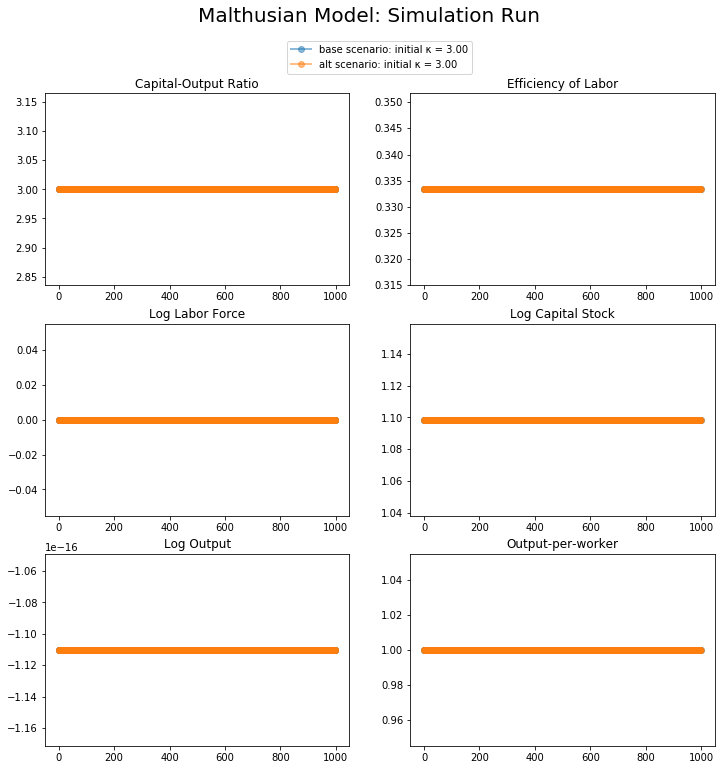In :
# TESTING THE MALTHUSIAN CLASS
#
# Adding idea stock growth fast enough that
# (with γ=2) population will double every
# 700 years
#
# Starting the economy at the h=0 Malthusian

import numpy as np
import matplotlib.pyplot as plt
%matplotlib inline

import delong_classes

T = 1000

m_base = delong_classes.malthusian(h=.0005)
m_base.scenario = "base scenario"
m_alt = delong_classes.malthusian(h=.0005)
m_alt.scenario = "alt scenario"

figcontents = {
(0,0):('κ','Capital-Output Ratio', False),
(0,1):('E','Efficiency of Labor', False),
(1,0):('L','Log Labor Force', True),
(1,1):('K','Log Capital Stock', True),
(2,0):('Y','Log Output', True),
(2,1):('y','Output-per-worker', False)
}

num_rows, num_cols = 3,2
fig, axes = plt.subplots(num_rows, num_cols, figsize=(12, 12))
for i in range(num_rows):
for j in range(num_cols):
for m in m_base, m_alt:
lb = f'{m.scenario}: initial κ = {m.initdata["κ"]:.2f}'
axes[i,j].plot(m.gen_seq(T, var = figcontents[i,j], log = figcontents[i,j]),'o-', lw=2, alpha=0.5, label=lb)
axes[i,j].set(title=figcontents[i,j])

#   global legend
axes[(0,0)].legend(loc='upper center', bbox_to_anchor=(1.1,1.3))
plt.suptitle('Malthusian Model: Simulation Run', size = 20)
plt.show()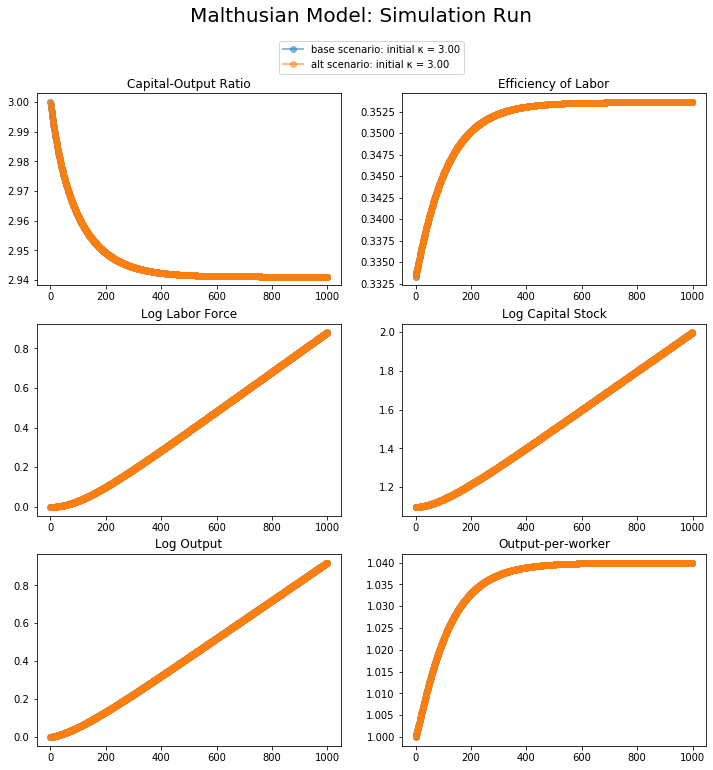Notice that the steady state is not affected by where we start.

### Assume useful ideas stopped developing since 250AD:¶

In :
import numpy as np
import matplotlib.pyplot as plt
%matplotlib inline

import delong_classes

m  = delong_classes.malthusian(h=0.0005, E=0.354, K=3.06)

# generate and store sequences before the change:
T1 = 250 # time before change
T2 = 250 # time after change

figcontents = {
(0,0):('κ','Capital-Output Ratio', False),
(0,1):('E','Efficiency of Labor', False),
(1,0):('L','Log Labor Force', True),
(1,1):('K','Log Capital Stock', True),
(2,0):('Y','Log Output', True),
(2,1):('y','Output-per-worker', False)
}

num_rows, num_cols = 3,2
fig, axes = plt.subplots(num_rows, num_cols, figsize=(12, 12))
for i in range(num_rows):
for j in range(num_cols):
for scenario in {'base', 'with shock'}:
seq = m.gen_seq(T1, var = figcontents[i,j], log = figcontents[i,j])
lb = f'{scenario}'
if scenario == 'with shock':
m.h = 0
seq = np.append(seq, m.gen_seq(T2, var = figcontents[i,j], log = figcontents[i,j], init = False))
axes[i,j].plot(seq,'o-', lw=2, alpha=0.5, label=lb)
axes[i,j].set(title=figcontents[i,j])

axes[(0,0)].legend(loc='upper center', bbox_to_anchor=(1.1,1.3))
plt.suptitle('Malthusian Model: Simulation Run with Idea Shock', size = 20)
plt.show()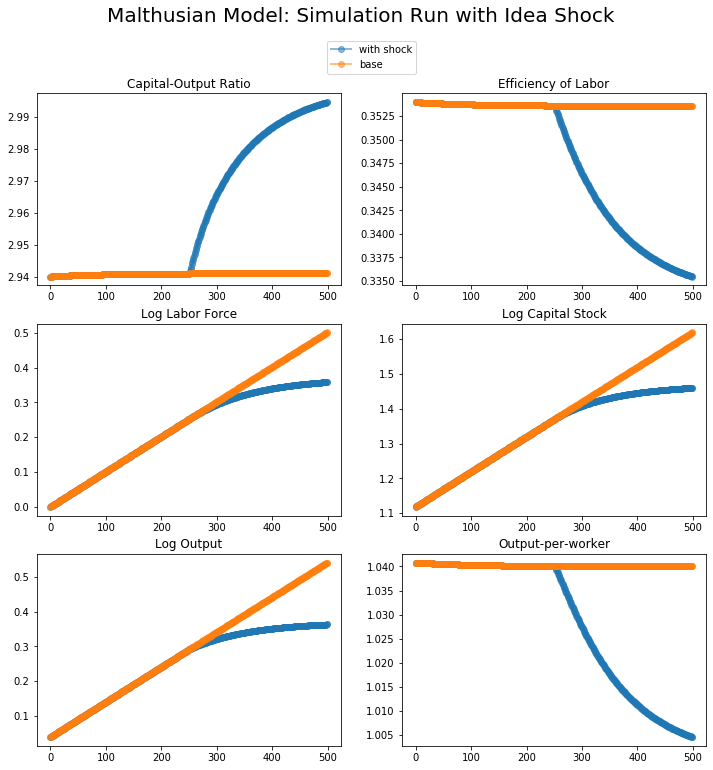In :
# THE COMING OF AN IMPERIAL PEACE

# φ = 1.25, s = 0.25

import numpy as np
import matplotlib.pyplot as plt

import delong_classes

m  = delong_classes.malthusian(h=0.0005, E=0.354, K=3.06)

# generate and store sequences before the change:
T1 = 250 # time before change
T2 = 250 # time after change

figcontents = {
(0,0):('κ','Capital-Output Ratio', False),
(0,1):('E','Efficiency of Labor', False),
(1,0):('L','Log Labor Force', True),
(1,1):('K','Log Capital Stock', True),
(2,0):('Y','Log Output', True),
(2,1):('y','Output-per-worker', False)
}

num_rows, num_cols = 3,2
fig, axes = plt.subplots(num_rows, num_cols, figsize=(12, 12))
for i in range(num_rows):
for j in range(num_cols):
for scenario in {'base', 'with shock'}:
seq = m.gen_seq(T1, var = figcontents[i,j], log = figcontents[i,j])
lb = f'{scenario}'
if scenario == 'with shock':
m.φ = 1.25
m.s = 0.25
seq = np.append(seq, m.gen_seq(T2, var = figcontents[i,j], log = figcontents[i,j], init = False))
axes[i,j].plot(seq,'o-', lw=2, alpha=0.5, label=lb)
axes[i,j].set(title=figcontents[i,j])

axes[(0,0)].legend(loc='upper center', bbox_to_anchor=(1.1,1.3))
plt.suptitle('Malthusian Model: Simulation Run with Idea Shock', size = 20)
plt.show()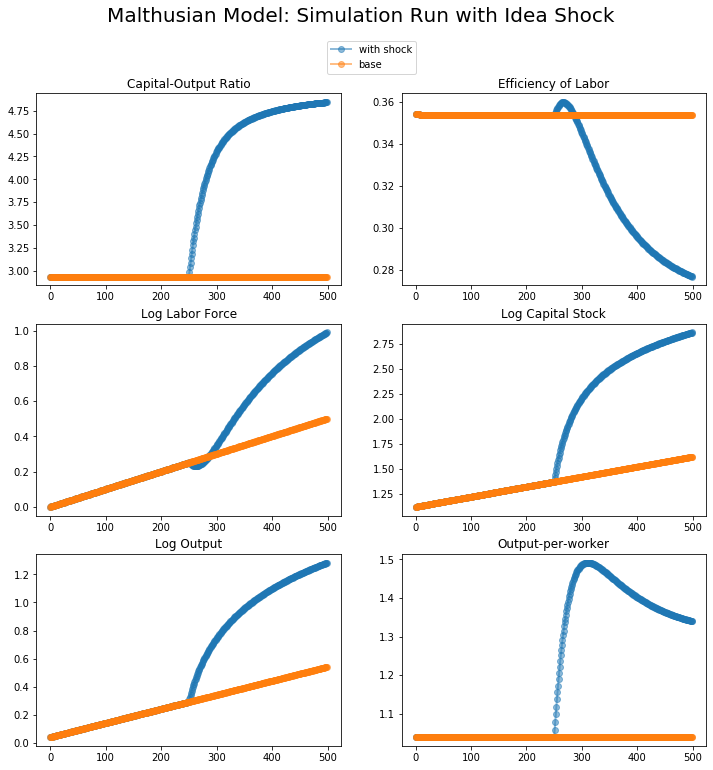In :
# THE COLLAPSE OF AN EMPIRE

# φ = 1.25, s = 0.25

import numpy as np
import matplotlib.pyplot as plt

import delong_classes

m  = delong_classes.malthusian(h=0.0005, E=0.27, K=6.5, φ = 1.25, s = 0.25)

# generate and store sequences before the change:
T1 = 250 # time before change
T2 = 250 # time after change

figcontents = {
(0,0):('κ','Capital-Output Ratio', False),
(0,1):('E','Efficiency of Labor', False),
(1,0):('L','Log Labor Force', True),
(1,1):('K','Log Capital Stock', True),
(2,0):('Y','Log Output', True),
(2,1):('y','Output-per-worker', False)
}

num_rows, num_cols = 3,2
fig, axes = plt.subplots(num_rows, num_cols, figsize=(12, 12))
for i in range(num_rows):
for j in range(num_cols):
for scenario in {'base', 'with shock'}:
seq = m.gen_seq(T1, var = figcontents[i,j], log = figcontents[i,j])
lb = f'{scenario}'
if scenario == 'with shock':
m.φ = 1.0
m.s = 0.15
seq = np.append(seq, m.gen_seq(T2, var = figcontents[i,j], log = figcontents[i,j], init = False))
axes[i,j].plot(seq,'o-', lw=2, alpha=0.5, label=lb)
axes[i,j].set(title=figcontents[i,j])

axes[(0,0)].legend(loc='upper center', bbox_to_anchor=(1.1,1.3))
plt.suptitle('Malthusian Model: Simulation Run with Idea Shock', size = 20)
plt.show()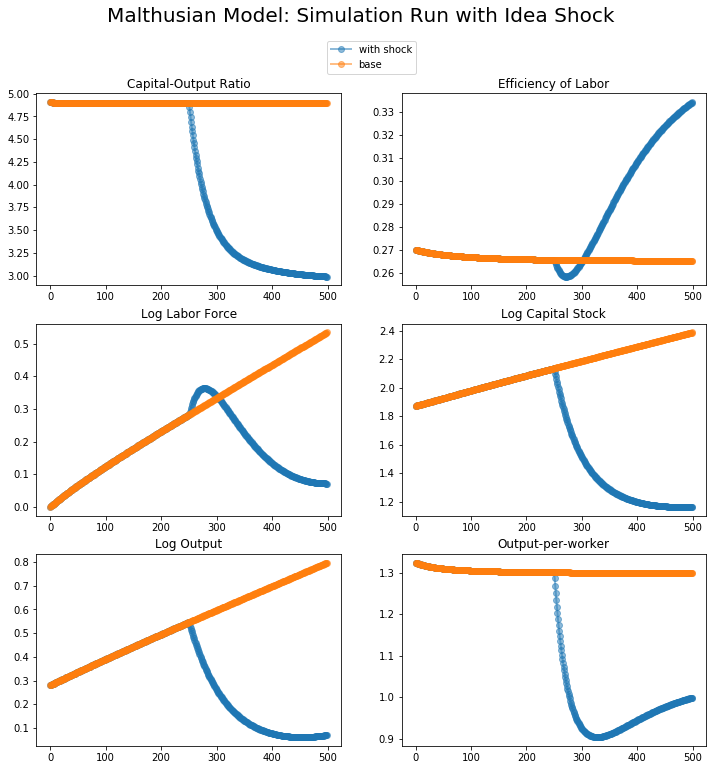### Consider a plague happened on 250AD:¶

The plague decreased the total population (and thus labor force) to 1/10.

In :
import numpy as np
import matplotlib.pyplot as plt

import delong_classes

m  = delong_classes.malthusian(h=0.0005, E=0.354, K=3.06)

# generate and store sequences before the change:
T1 = 250 # time before change
T2 = 250 # time after change

figcontents = {
(0,0):('κ','Capital-Output Ratio', False),
(0,1):('E','Efficiency of Labor', False),
(1,0):('L','Log Labor Force', True),
(1,1):('K','Log Capital Stock', True),
(2,0):('Y','Log Output', True),
(2,1):('y','Output-per-worker', False)
}

num_rows, num_cols = 3,2
fig, axes = plt.subplots(num_rows, num_cols, figsize=(12, 12))
for i in range(num_rows):
for j in range(num_cols):
for scenario in {'base', 'with plague'}:
seq = m.gen_seq(T1, var = figcontents[i,j], log = figcontents[i,j])
lb = f'{scenario}'
if scenario == 'with plague':
m.L = 2/3*m.L
m.E = m.E*(2/3)**(-1/m.γ)
seq = np.append(seq, m.gen_seq(T2, var = figcontents[i,j], log = figcontents[i,j], init = False))
axes[i,j].plot(seq,'o-', lw=2, alpha=0.5, label=lb)
axes[i,j].set(title=figcontents[i,j])

axes[(0,0)].legend(loc='upper center', bbox_to_anchor=(1.1,1.3))
plt.suptitle('Malthusian Model: Simulation Run with Plague', size = 20)
plt.show()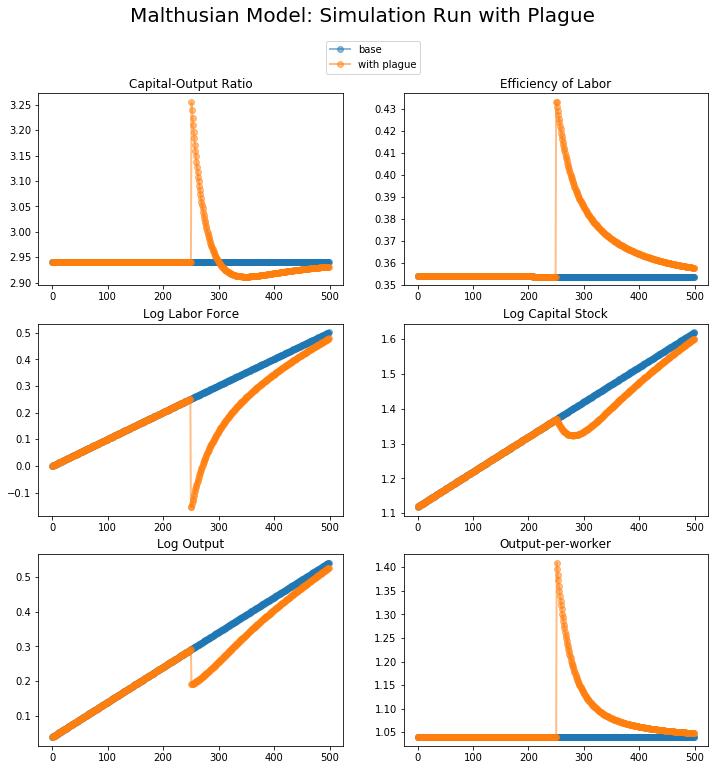To summarize, the shock on idea development will change the steady state and the development path, while a plague would not change the steady state.

### Shocks to the System... ¶

• A plague, pushing $L_t$ down substantially below $L_t^{*mal}$...
• A breakdown (or buildup) of law-and-order, raising incentives to save and invest, and so raising $s$...
• Invaders and other raiders, raising $\delta$...
• A speed-up or slowdown in innovation, raising or lowering $h$...
• Large-scale destruction of the societal web, lowering $H$...
• An increased rate of death from disease or violence, raising or lowering $Y^{sub}$...

## A Solow-Malthus Model of Ancient Economies ¶### Catch Our Breath—Further Notes:¶

In [ ]: